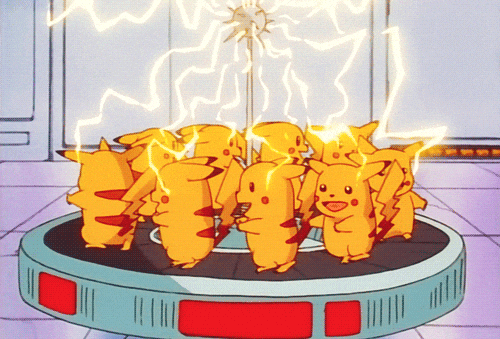# Intro to Video AnalysisWe’ll be doing some video analysis of a rolling ball in Capstone today. Woot woot.

For the analysis of the video that you make with your partner:

• Look at the horizontal position vs time graph.
• What is the general shape of this graph? What does that mean?
• What is the slope of the line? What does that mean?
• Look at the vertical position vs time graph.
• What is the general shape of this graph? What does that mean?
• Look at the vertical velocity vs time graph.
• What is the slope of this graph? What does that mean?

# Motion Terminology Self TestPlay the Motion Terminology Self-Test! You must get 10 answers correct in a row. If you get one wrong, you will have to restart the whole thing.

Ok, now try this one: Finding Velocity from Position Graph

# Find the Mass of Peter Griffin

Use this video below to find the mass of Peter Griffin:

# ROTATION LAB!!!# CSI ProjectilesClick here to go to the lab activity for today. Type your answers into that document and share it with me when you finished it.

When you are done with the CSI activity, complete the two short tasks below. Pay attention to the units and make sure you read the question so you know what you are supposed to answer. Screenshot your success and share it with me.

# Throwback Thursday (on a Wednesday)Try this old example of finding the net force of three forces at different angles.

https://www.thephysicsaviary.com/Physics/APPrograms/NetForceHard/index.html

Almost like a Quadrant-tester for cell F7:

=IF(AND(D7>0,E7>0),1,IF(AND(D7<0,E7>0),2,IF(AND(D7<0,E7<0),3,IF(E7=0,0,4))))

Updated formula for the resultant angle in cell C7:

=IF(F7=0,0,IF(OR(F7 = 2, F7 = 3), 180 + DEGREES(ATAN(E7/D7)), IF(F7=4, 360 + DEGREES(ATAN(E7/D7)), DEGREES(ATAN(E7/D7)))))

# Circuit Challenges

Watt’s Up!!! It’s FRIDAY!Try these circuit challenges to test your circuit skills.

# Simple Harmonic MotionIT”S FRIDAY!!!!!

Working with a buddy, try these two problems: